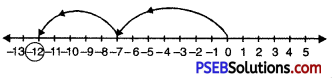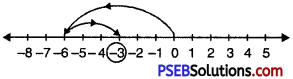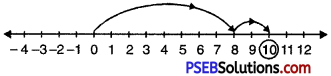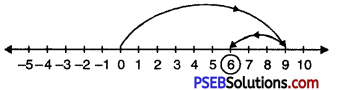# PSEB 6th Class Maths Solutions Chapter 4 Integers Ex 4.3

Punjab State Board PSEB 6th Class Maths Book Solutions Chapter 4 Integers Ex 4.3 Textbook Exercise Questions and Answers.

## PSEB Solutions for Class 6 Maths Chapter 4 Integers Ex 4.3

1. Fill the suitable integer in box:

Question (i)
(a) 2 + _ = 0
(b) _ + 11 =0
(c) -5 + _ = o
(d) _ + (-9) = 0
(e) 3 + _ = 0
(f) _ + 0 = 0.
Solution:
(a) 2 + -2 = 0
(b) -11 + 11 =0
(c) -5 + 5 = o
(d) 9 + (-9) = 0
(e) 3 + (-3) = 0
(f) 0 + 0 = 0.2. Subtract using number line:

Question (a)
5 from -7
Solution:
(-7) – 5Hence , -7 – 5 = -12

Question (b)
-3 from -6
Solution:
-6 – (-3)
= -6 + (additive inverse of -3)
= -6 + (3)Hence, -6 + 3 = -3

Question (c)
-2 from 8
Solution:
8 – (-2)
= 8 + (additive inverse of -2)
= 8 + (2)Hence, 8 + 2 = 10Question (d)
3 from 9.
Solution:
9 – 3Hence, 9 – 3 = 6

3. Subtract without using number line:

Question (a)
-6 from 16
Solution:
16 – (-6)
= 16 + (6) = 16 + 6
= 22

Question (b)
-51 from 55
Solution:
55 – (-51)
= 55 + (51) = 55 + 51
= 106

Question (c)
75 from -10
Solution:
-10 – 75
= -(10 + 75)
= -85Question (d)
-31 from -47.
Solution:
-47 – (-31)
= -47 + 31 = -(47 – 31)
= -16

4. Find:

Question (a)
35 – (20)
Solution:
= (35 – 20)
= 15

Question (b)
(-20) – (13)
Solution:
= -(20 + 13)
= -33

Question (c)
(-15) – (-18)
Solution:
= (-15) + (18)
= 3Question (d)
72 – (90)
Solution:
= -(90 – 72)
= -18

Question (e)
23 – (-12)
Solution:
= 23 + (12)
= 23 + 12
= 35

Question (f)
(-32) – (-40).
Solution:
= 40 – 32
= 8

5. Simplify:

Question (a)
2 – 4 + 6 – 8 – 10
Solution:
= 2 + 6 – 4 – 8 – 10
= 2 + 6 -(4 + 8 + 10)
= 8 – 22
= -14

Question (b)
4 – 2 + 2 – 4 – 2 + 2
Solution:
= 4 + 2 + 2 – 2 – 4 – 2
= 8 – 8
= 0Question (c)
4 – (-9) + 7 – (-3)
Solution:
=4 + 9 + 7 + 3
= 23

Question (d)
(-7) + (-19) + (-7).
Solution:
= -(7 + 19 + 7)
= – 33